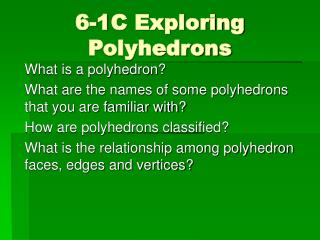# 6-1C Exploring Polyhedrons - PowerPoint PPT PresentationDownload Presentation6-1C Exploring Polyhedrons

Download Presentation## 6-1C Exploring Polyhedrons

- - - - - - - - - - - - - - - - - - - - - - - - - - - E N D - - - - - - - - - - - - - - - - - - - - - - - - - - -
##### Presentation Transcript

1. 6-1C Exploring Polyhedrons What is a polyhedron? What are the names of some polyhedrons that you are familiar with? How are polyhedrons classified? What is the relationship among polyhedron faces, edges and vertices?

2. Definition A polyhedron is a solid whose faces are polygons. Faces are named in the same way as polygons. What are some polyhedrons that your are familiar with? Cubes, pyramids, and rectangular solids Look at the figure on page410 in your book. Name a pentagonal face.

3. Try It Page 410 • Polygons are classified by the number of sides they have. • Polyhedrons are classified by the number of faces they have. • See page 410

4. Face Facts p. 411 4 4 6 a. b. c. d. e. 8 12 6 6 12 8 7 15 10 5 6 9 Is there a relationship among the faces, vertices & edges?

5. Euler’s Formula For any polyhedron, the relationship among the number of faces (F), vertices (V), and edges (E) is F + V − E = 2

6. Leonhard Euler • Lived 1707-1783 • Swiss mathematician • Proved the formula showing the relationship among the faces, vertices and edges of polyhedron.

7. What is a polyhedron? A polyhedron is a solid whose faces are polygons. What are the names of some polyhedrons that you are familiar with? Cubes, pyramids, and rectangular solids. • How are polyhedrons classified? Polyhedrons are classified by the number of faces they have. • What is the relationship among polyhedron faces, edges and vertices? F + V − E = 2

8. Assignment 6-1C Page 412, 1-8, 11-13, 16-18